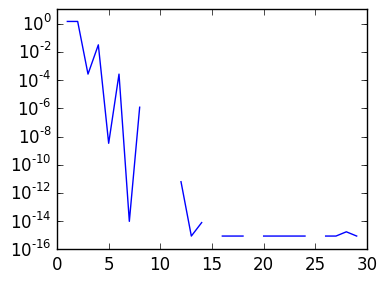Numerical integration (which is also called quadrature) refers to approximating integrals by sums. A quadrature rule is the pair of nodes $(x_1,\ldots,x_n)$ and weights $(w_1,\ldots,w_n)$ so that

$$\int_a^b f(x) dx \approx \sum_{k=1}^n w_k f(x_k)$$

In this lecture, we will focus on integrals over $[0,1]$:

$$\int_0^1 f(x) dx$$

We will also use $[0,2\pi)$ when we wish to emphasize periodicity:

$$\int_0^{2\pi} f(\theta) d\theta$$

## Right-hand rule¶

We begin with the simplest method the right-hand rule. This is the choice of nodes $x_k = kh$ and weights $w_k = h$ for $h \triangleq {1 \over n}$.

This rule corresponds to integrating rectangles which match the right-hand value in each panel, consisting of $x$ between $x_k$ and $x_{k+1}$. We will depict this now.

Consider a simple function on $[0,1]$:

In :
using PyPlot

f=x->cos(3x).*exp(x)+1

g=linspace(0.,1.,1000)  # the plotting grid, much finer than the quadrature nodes
plot(g,f(g));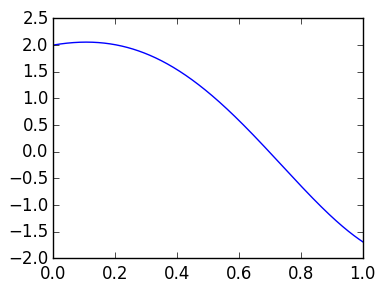Our quadrature nodes are $(x_1,\ldots,x_n)=[h,2h,\ldots,1]$, as plotted here:

In :
n=3
h=1/n
x=linspace(h,1.,n)   # creates n evenly spaced nodes between h and 1

scatter(x,zeros(x))
axis([0.,1.,-1.,1.]);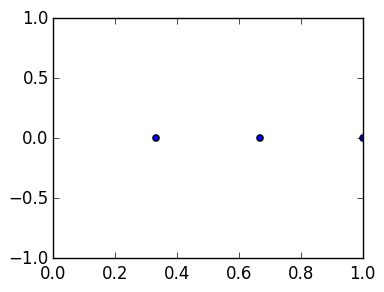/usr/local/lib/python2.7/site-packages/matplotlib/collections.py:590: FutureWarning: elementwise comparison failed; returning scalar instead, but in the future will perform elementwise comparison
if self._edgecolors == str('face'):


To plot the rectangles, we create a function s(vals,x,t) that plots a piecewise step function which equals vals[k] between x[k-1] and x[k]:

In :
function s(vals::AbstractVector,x::AbstractVector,t::Number)
n=length(x)

if 0 ≤ t ≤ x
return vals
end

for k=1:n-1
# check each panel one by one
if x[k] ≤ t ≤ x[k+1]
return vals[k+1]
end
end

return zero(typeof(vals))  # return 0. of the same type as vals[k]
end

Out:
s (generic function with 1 method)

We can plot the rectangle approximation versus the true function:

In :
n=5
h=1/n
x=linspace(h,1.,n)

plot(g,f(g))
plot(g,Float64[s(f(x),x,t) for t in g])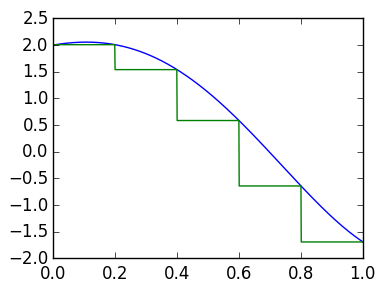Out:
1-element Array{Any,1}:
PyObject <matplotlib.lines.Line2D object at 0x313244bd0>

The quadrature rule is given by the approximation

sum(f(x)*h)   # sum([v1,v2,v3]) = v1+v2+v3


We compare with an approximation of the true integrand, using quadgk:

In :
ex,err=quadgk(f,0.,1.)

n=1000000
h=1/n
x=linspace(h,1.,n)

sum(f(x)*h)-ex

Out:
-1.8455397103878113e-6

We see that our method is much slower than quadgk: one should not use this method in practice!

In :
@time sum(f(x)*h)

  0.071964 seconds (169 allocations: 38.158 MB, 11.53% gc time)

Out:
0.7459714791406495
In :
@time quadgk(f,0.,1.)

  0.000036 seconds (165 allocations: 2.922 KB)

Out:
(0.7459733246803599,1.0242917625191694e-12)

We estimate the convergence rate using a loglog plot. The slope of a loglog plot tells us the rate $\alpha$ such that the error behaves like $O(n^{-\alpha})$.

Since the slopes match, the following plot is indication that the error decays like $O(n^{-1})$. We will prove this next lecture.

In :
ns=round(Int,logspace(1,5,100))   # create 100 logarithmically spaced n's between 10^1 and 10^5

err=zeros(length(ns))  # we will fill this Vector with the errors

for k=1:length(ns)
n=ns[k]
h=1/n
x=linspace(h,1.,n)

err[k]=abs(sum(f(x)*h)-ex    )
end

loglog(ns,err)    # The blue curve
loglog(ns,1./ns)  # The green curve.  Since the slope appears to match the green curve, we
# conjecture that the blue curve is also O(1/n)
loglog(ns,1./ns.^2)  # The red curve. We also plot something which decays faster so we
#  can see that the slope is clearly different;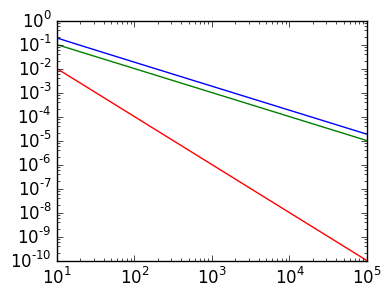# Trapezium rule¶

The trapezium rule approximates by an affine function in each panel, which is then integrated exactly. This leads to the approximation

$$\int_0^1 f(x) dx \approx h\left({f(x_0) \over 2} + \sum_{k=1}^{n-1} f(x_k) + {f(x_n) \over 2} \right)$$

where again $x_k ={kh}$. (Note: we will sometimes start our indices from zero when it simplifies notation. This is therefore an $n+1$ point quadrature rule.)

This expression follows since in each panel we have the affine approximation (using the first panel as example):

$$f(x) \approx f(0) + x {f(h) - f(0) \over h}$$

which equals $f$ at $x_k$ and $x_{k+1}$. Integrating this approximation exactly gives us

$$\int_0^h \left(f(0) + x {f(h) - f(0) \over h}\right) dx = f(0)h + {h^2 \over 2} {f(h) - f(0) \over h} = h {f(0) + f(h) \over 2}$$

We thus have

\begin{align*} \int_0^1 f(x) dx &\approx \sum_{k=1}^n \int_0^1 \left(f(x_{k-1}) + (x-x_{k-1}) {f(x_k) - f(x_{k-1}) \over h}\right) dx \cr &= h\sum_{k=1}^n {f(x_{k-1}) + f(x_k) \over 2} \cr &= h\left({f(x_0) \over 2} + \sum_{k=1}^{n-1} f(x_k) + {f(x_n) \over 2} \right) \end{align*}

We will depict the approximation. Consider again a simple function. Now plot will plot the approximation by trapeziums:

In :
plot(g,f(g))

n=3

h=1/n
x=linspace(0.,1.,n+1)  # the grid

plot(x,f(x));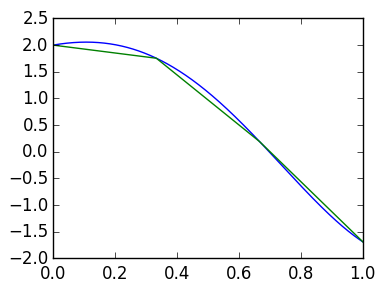The function trap(f,n) calculates the trapezoidal rule between 0 and 1 with n panels:

In :
ex,err=quadgk(f,0.,1.)

n=1000

function trap(f,n)
h=1/n
x=linspace(0.,1.,n+1)

v=f(x)  # assume f can be evaluated on Vectors.  Otherwise, use map(f,x)
h/2*v+sum(v[2:end-1]*h)+h/2*v[end]
end

trap(f,10000)-ex   # the error is smaller than before.

Out:
-4.0349085184132605e-9

Let's compare the error in trap to the right-hand rule. The following shows that the error is $O(n^{-2})$:

In :
ns=round(Int,logspace(1,5,100))

err=zeros(length(ns))
errT=zeros(length(ns))

for k=1:length(ns)
n=ns[k]
h=1/n
x=linspace(h,1.,n)

err[k]=abs(sum(f(x)*h)-ex    )
errT[k]=abs(trap(f,n-1)-ex    )
end

loglog(ns,err)       # blue curve
loglog(ns,errT)      # green curve

loglog(ns,1./ns)     # red curve
loglog(ns,1./ns.^2)  # aqua curve;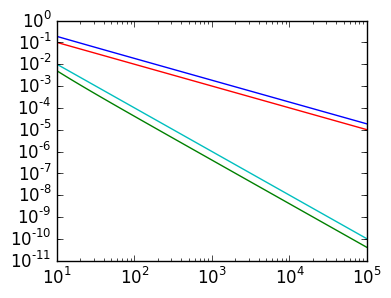# Periodic functions¶

One last thing, which should come as a suprise. Consider now a periodic function (on $[0,2π)$):

In :
f=θ -> cos(cos(θ))

g=linspace(0.,2π,1000)

plot(g,f(g));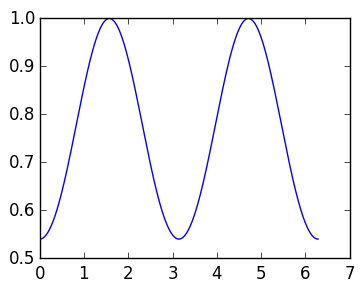Approximating the functions by trapeziums is still very inaccurate:

In :
n=4
θ=linspace(0.,2π,n+1)

plot(θ,f(θ))
plot(g,f(g));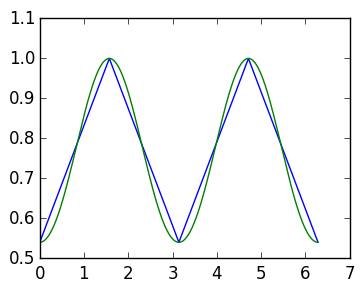The trapθ function gives the trapezium rule on [0,2π), which is just the original trapezium rule scaled by 2π.

In :
function trapθ(f,n)
h=2π/n
x=linspace(0.,2π,n+1)

v=f(x)
h/2*v+sum(v[2:end-1]*h)+h/2*v[end]
end;


Amazingly, the error is tiny!

In :
ex=quadgk(f,0.,2π)
trapθ(f,16)-ex

Out:
-8.881784197001252e-16

This is despite the fact that the trapezoids are still no where near the curve:

In :
n=16
θ=linspace(0.,2π,n+1)

plot(θ,f(θ))
plot(g,f(g));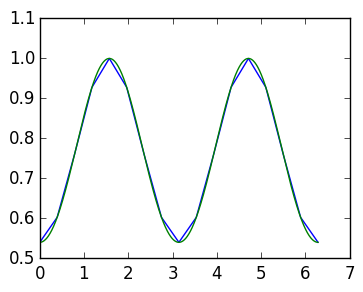We can plot the error with semilogy, to see that the error is actually going down exponentially fast:

In :
ns=1:30

errT=zeros(length(ns))

for k=1:length(ns)
errT[k]=abs(trapθ(f,k)-ex    )
end

semilogy(ns,errT);